# Precalculus : Graph the Sine or Cosine Function

## Example Questions

### Example Question #1 : Graph The Sine Or Cosine Function

Which of the following functions has a y-intercept of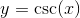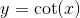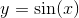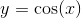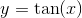Explanation:

The y-intercept of a function is found by substituting. When we do this to each, we can determine the y-intercept. Don't forget your unit circle!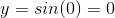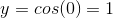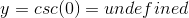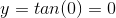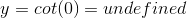Thus, the function with a y-intercept ofis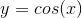### All Precalculus Resources